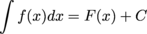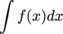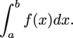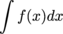We are here with you hands in hands to facilitate your learning & don't appreciate the idea of copying or replicating solutions. Read More>>

#www.vustudents.ning.com

 www.bit.ly/vucodes + Link For Assignments, GDBs & Online Quizzes Solution www.bit.ly/papersvu + Link For Past Papers, Solved MCQs, Short Notes & More

Dear Students! Share your Assignments / GDBs / Quizzes files as you receive in your LMS, So it can be discussed/solved timely. Add Discussion

# Graded Discussion Board for Math 101 Opening Date feb 6,2013 and closing date feb 7,2013

Discuss the role of constant of integration in indefinite integrals, why we don't involve it in definite integrals? (Be precise)

Opening Date: Feb 6, 2013 at 12:01 AM

Closing Date: Feb 7, 2013 at 11:59 PM

Instructions

(1) Post your comments only on the concern Graded MDB forum and not on regular MDB forum.

(2) Write your comments in the plain text and avoid math type symbols and figures/graphs/images as these will not appear on the board.

(3) Zero marks will be given to irrelevant comments or to those which are copied from website or any other source.

(6) Due date will not be extended.

(7) No description will be accepted through e-mail.

+ How to Join Subject Study Groups & Get Helping Material?

+ How to become Top Reputation, Angels, Intellectual, Featured Members & Moderators?

+ VU Students Reserves The Right to Delete Your Profile, If?

Views: 1558

.

+ http://bit.ly/vucodes (Link for Assignments, GDBs & Online Quizzes Solution)

+ http://bit.ly/papersvu (Link for Past Papers, Solved MCQs, Short Notes & More)

### Replies to This Discussion

thaaaaaaaaaaaaaaaanx a lot bro

Idea..

You can approximate the area under a curve by adding up right, left, or midpoint rectangles. To find an exact area, you need to use a definite integral.
When you approximate the area under a curve, the tops of the rectangles form a saw tooth shape that doesn’t fit perfectly along the smooth curving function. So, to find the exact area under a curve using rectangles, you’d need to find the area of an infinite number of infinitely thin rectangles whose “tops” do perfectly fit the curve. Now, you can’t really use an infinite number of rectangles, but with the fantastic invention of limits, this is sort of what happens.
Here’s the “simple” definition of the definite integral that’s used to compute exact areas. It’s based on the limit of a Riemann sum of right rectangles. The exact area under a curve between a and b is given by the definite integral, which is defined as follows:

thaaaaaaaaaaaaanx broIn the equationthe letter C is called the constant of integration. The function f ( x ) is still called the integrand, like it was for the definite integral.

The indefinite integraland the general solution to the differential equation both describe the same thing: the collection of all functions with derivative f ( x ). Whether we're working in the context of integrals or of differential equations, we use the constant + Cto describe all antiderivatives or all solutions at once.

A definite integral is an integral of the formThe integral sign has limits of integration. A definite integral is a number. There's no need to write + C.

An indefinite integral is an integral of the form.

There are no limits of integration on the integral sign. This indefinite integral is the family of all antiderivatives of f ( x ). Remember to write + C!Nice guys keep it up

In definite integrals we don't involve constant of integration because definite integral gives us a definite value at the point of calculation .In it we use two limits as a and b which is area under the curve .Definite integral has limits and numbers above and below the integral sign but in indefinite integrals which is unlimited or boundless we always add an unknown constant which is constant of integration because the derivative of F(x)+C is equal to the derivative of F(x).

thanks aloooooooooooooooooot bro.

## Latest Activity+¢αяєℓєѕѕ gιяℓ posted a video

### Ik Khawab Sunawan - Rahat Fateh Ali Khan - Na'at Album "Ya Nabi"Rana Ali posted a video